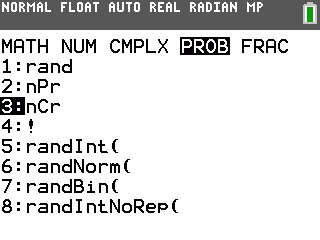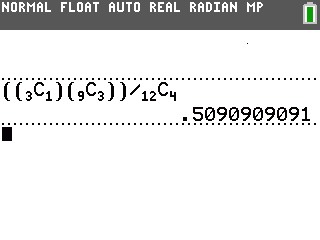# Knowledge Base

## Solution 34877: Calculating Hypergeometric Probability Distributions on the TI-84 Plus CE and TI-84 Plus C Silver Edition.

### How do I calculate a hypergeometric probability distribution on the TI-84 Plus CE and TI-84 Plus C Silver Edition?

There is no pre-defined function in the calculator that will find the statistical values for a hypergeometric probability distribution. However, users can enter the formula to find the results for this type of distribution into the calculator. The instructions below describe how to find P(1) of a hypergeometric probability distribution.

Example: Find P(1) when N = 12, n = 4, and k = 3

Since P(x) = {C(k, x) × C(N-k, n-x)} ÷ C(N, n)

With substitution, P(1) = {C(3, 1) × C(12-3, 4-1)} ÷ C(12, 4)

So the values should be entered using the following steps:

1) Press [(] [(] .
2) Press [MATH] [►] [►] [►].
3) Press  to select 3:nCr.4) Press  [►] [)].
5) Press [(] .
6) Press [MATH] [►] [►] [►].
7) Press  to select 3:nCr.
8) Press  [►].
9) Press [)] [)] [÷]  .
10) Press [MATH] [►] [►] [►].
11) Press  to select 3:nCr.
12) Press  [ENTER].Please see the TI-84 Plus CE and TI-84 Plus C Silver Edition guidebooks for additional information.